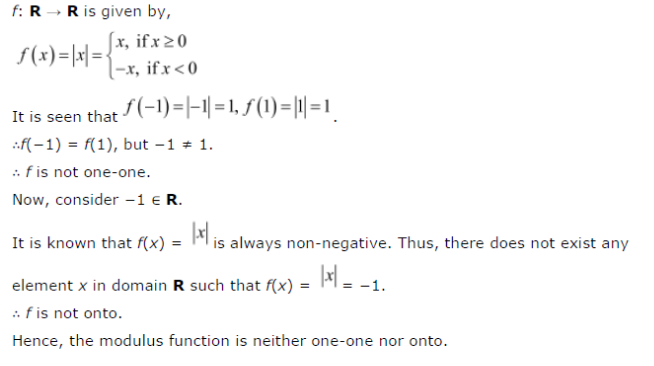# Show that the Modulus Function f : R → R , given by f(x) = |x|, is neither one-one nor onto,

Show that the Modulus Function f : R → R , given by f(x) = |x|, is neither one-one nor onto, where is x if x is positive or 0 and |x| is -1, if x is negative.